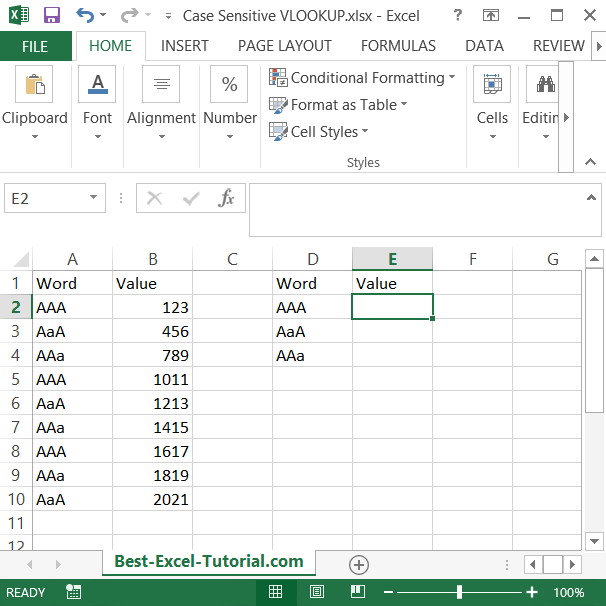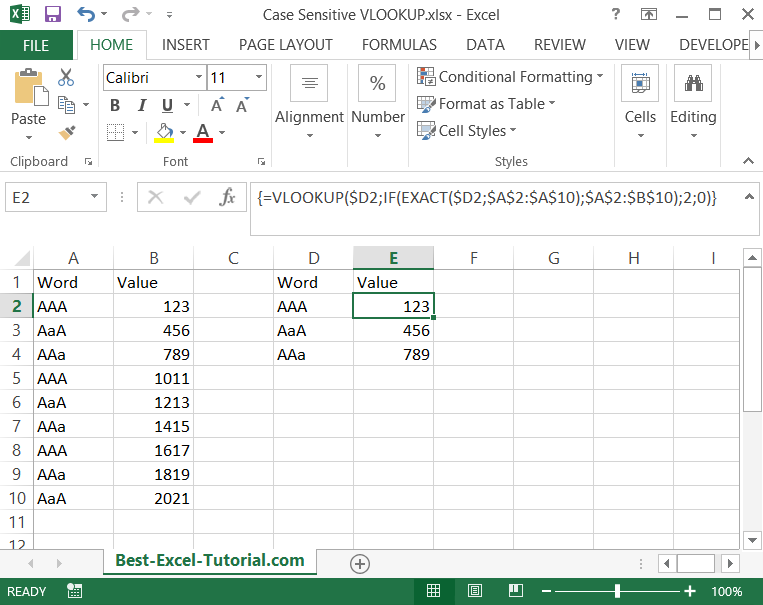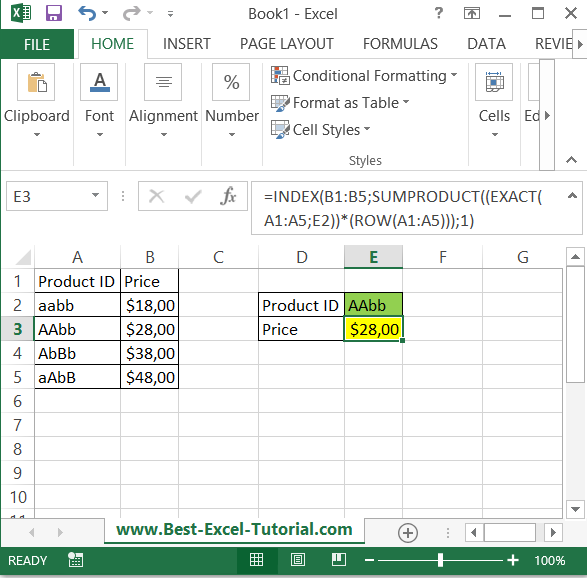#### Case-Sensitive VLOOKUP

In this Excel lesson you will teach yourself how to create a case-sensitive vlookup. This is a clever way to solve many problems caused by caps lock keyboard button.

## Case-sensitive vlookup

By default, VLOOKUP is not case sensitive, meaning that it treats uppercase and lowercase letters as the same. For example, if you use VLOOKUP to search for the value “apple” in a table that contains “Apple”, it will return the value in the same row.

Vlookup function doesn’t care about case-sensitivity. So what to do when you do?

This is an example:The task is to vloookup the value to the word. Formula should care about case-sensitivity.

The solution is this array formula:

=VLOOKUP(value,IF(EXACT(value,column),table),column_number,0)

0 here means the exact match for the vlookup function.Formula in the example is:

{=VLOOKUP(\$D2,IF(EXACT(\$D2,\$A\$2:\$A\$10),\$A\$2:\$B\$10),2;0)}

Please be aware that it is an array formula so you need to use CTRL + SHIFT + ENTER keyboard shortcut. That’s why there are braces around the formula. They appears after you typed CTRL + SHIFT + ENTER.

Keep in mind that this approach requires you to use additional columns and functions, which may not be as efficient as a simple VLOOKUP formula. However, it gives you more control over the matching process and allows you to perform case-sensitive lookups when necessary.

## Case-sensitive lookup

The problem is that your data differ only by case so capitalization is important for you. That’s why you need case sensitive lookup. Let’s construct a formula which will solve your problem. INDEX formula suits the best. Syntax here will be:

=INDEX(value_you_need, row_number, column_number)

• value_you_need is a data range from your data table
• row_number – this is hard to get. You have to use EXACT function for case sensitive function
• column_number is always 1

In this example you need the Price and you have Product IDs. The formula you need is:

=INDEX(B1:B5;SUMPRODUCT((EXACT(A1:A5;E2))*(ROW(A1:A5)));1)## Case-sensitive hlookup and xlookup

In Microsoft Excel, you can perform a case-sensitive HLOOKUP by using a combination of functions. HLOOKUP is similar to VLOOKUP, but it searches for a value in the top row of a table and returns a value in the same column from a row below.

Similarly, the XLOOKUP function, which is available in newer versions of Excel, can also be used to perform a case-insensitive lookup.

To perform a case-sensitive XLOOKUP in Excel, you can use the EXACT function with the XLOOKUP function.

By using these formulas together, you can perform a case-sensitive HLOOKUP or XLOOKUP in Excel. Note that the XLOOKUP function is only available in newer versions of Excel, so if you are using an older version, you will need to use the HLOOKUP method described earlier.